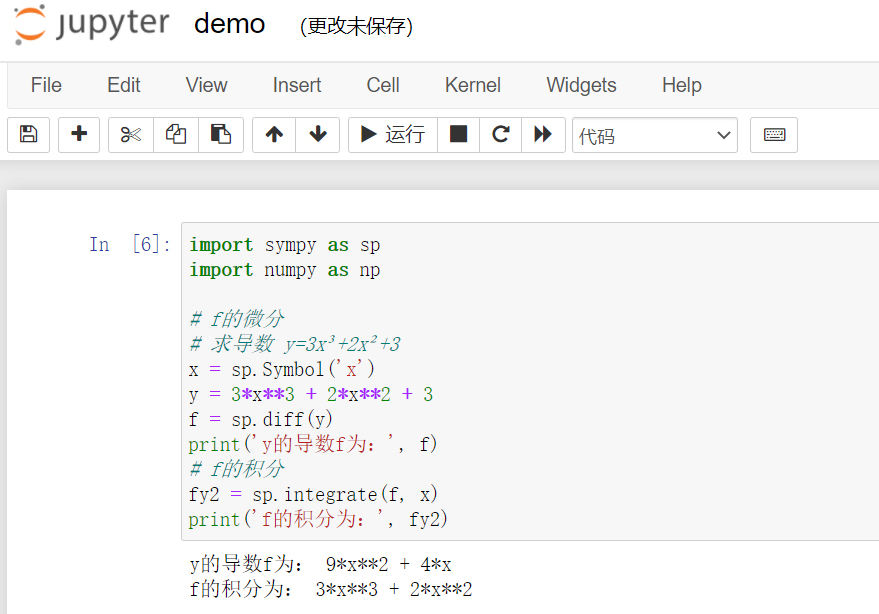﻿ 导数微分积分三者关系 – 蒋智昊的博客# 导数微分积分三者关系

1、导数是指 函数图像在某一点处的斜率，是纵坐标增量Δy和横坐标增量Δx在Δx>0时的比值。
2、微分是指 函数图像在某一点处的切线在横坐标取得增量Δx以后，纵坐标取得的增量，一般表示为dy。
3、积分是微分的逆运算，即知道了函数的导函数，反求原函数。

`pip install sympy -i https://pypi.tuna.tsinghua.edu.cn/simple/````import sympy as sp
import numpy as np

# f的微分
# 求导数 y=3x³+2x²+3
x = sp.Symbol('x')
y = 3*x**3 + 2*x**2 + 3
f = sp.diff(y)
print('y的导数f为：', f)

# f的积分
fy2 = sp.integrate(f, x)
print('f的积分为：', fy2)
```

PyCharm如下：jupyter notebook如下：## 3条评论

1. 博主你好，我在我的环境下运行代码提示：NameError: name ‘x’ is not defined

york
1. import sympy as sp
import numpy as np

# f的微分
# 求导数 y=3x³+2x²+3
x = sp.Symbol(‘x’)
y = 3 * x ** 3 + 2 * x ** 2 + 3
f = sp.diff(y)
print(‘y的导数f为：’, f)

# f的积分
fy2 = sp.integrate(f, x)
print(‘f的积分为：’, fy2)

蒋智昊
2. jupyter notebook代码复制的时候少了一句。
# 变量的定义
x = sp.Symbol(‘x’)

蒋智昊